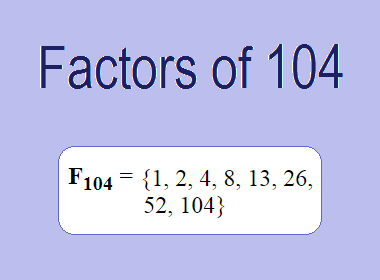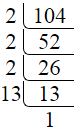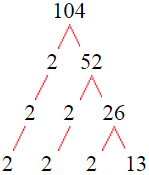# Factors of 104The factors of 104 are 1, 2, 4, 8, 13, 26, 52, and 104 i.e. F104 = {1, 2, 4, 8, 13, 26, 52, 104}. The factors of 104 are all the numbers that can divide 104 without leaving a remainder.

We can check if these numbers are factors of 104 by dividing 104 by each of them. If the result is a whole number, then the number is a factor of 104. Let's do this for each of the numbers listed above:

·        1 is a factor of 104 because 104 divided by 1 is 104.

·        2 is a factor of 104 because 104 divided by 2 is 52.

·        4 is a factor of 104 because 104 divided by 4 is 26.

·        8 is a factor of 104 because 104 divided by 8 is 13.

·        13 is a factor of 104 because 104 divided by 13 is 8.

·        26 is a factor of 104 because 104 divided by 26 is 4.

·        52 is a factor of 104 because 104 divided by 52 is 2.

·        104 is a factor of 104 because 104 divided by 104 is 1.

## How to Find Factors of 104?

1 and the number itself are the factors of every number. So, 1 and 104 are two factors of 104. To find the other factors of 104, we can start by dividing 104 by the numbers between 1 and 104. If we divide 104 by 2, we get a remainder of 0. Therefore, 2 is a factor of 104. If we divide 104 by 3, we get a remainder of 2. Therefore, 3 is not a factor of 104.

Next, we can check if 4 is a factor of 104. If we divide 104 by 4, we get a remainder of 0. Therefore, 4 is also a factor of 104. We can continue this process for all the possible factors of 104.

Through this process, we can find that the factors of 104 are 1, 2, 4, 8, 13, 26, 52, and 104. These are the only numbers that can divide 104 without leaving a remainder.

********************

********************

## Properties of the Factors of 104

The factors of 104 have some interesting properties. One of the properties is that the sum of the factors of 104 is equal to 210. We can see this by adding all the factors of 104 together:

1 + 2 + 4 + 8 + 13 + 26 + 52 + 104 = 210

Another property of the factors of 104 is that the prime factors of 104 are 2, and 13 only.

## Applications of the Factors of 104

The factors of 104 have several applications in mathematics. One of the applications is in finding the highest common factor (HCF) of two or more numbers. The HCF is the largest factor that two or more numbers have in common. For example, to find the HCF of 104 and 78, we need to find the factors of both numbers and identify the largest factor they have in common. The factors of 104 are 1, 2, 4, 8, 13, 26, 52, and 104. The factors of 78 are 1, 2, 3, 6, 13, 26, 39, and 78. The largest factor that they have in common is 26. Therefore, the HCF of 104 and 78 is 26.

Another application of the factors of 104 is in prime factorization. Prime factorization is the process of expressing a number as the product of its prime factors. The prime factors of 104 are 2, and 13, since these are the only prime numbers that can divide 104 without leaving a remainder. Therefore, we can express 104 as:

104 = 2 × 2 × 2 × 13

We can do prime factorization by division and factor tree method also. Here is the prime factorization of 104 by division method,104 = 2 × 2 × 2 × 13

Here is the prime factorization of 104 by the factor tree method,104 = 2 × 2 × 2 × 13

## Conclusion

The factors of 104 are the numbers that can divide 104 without leaving a remainder. The factors of 104 are 1, 2, 4, 8, 13, 26, 52, and 104. The factors of 104 have some interesting properties, such as having a sum of 210. The factors of 104 have several applications in mathematics, such as finding the highest common factor and prime factorization.